# Tag: nim-multiplication

Last time we introduced the game of transfinite Nimbers and asked a winning move for the transfinite game with stones a at position $~(2,2)$, b at $~(4,\omega)$, c at $~(\omega+2,\omega+3)$ and d at position $~(\omega+4,\omega+1)$.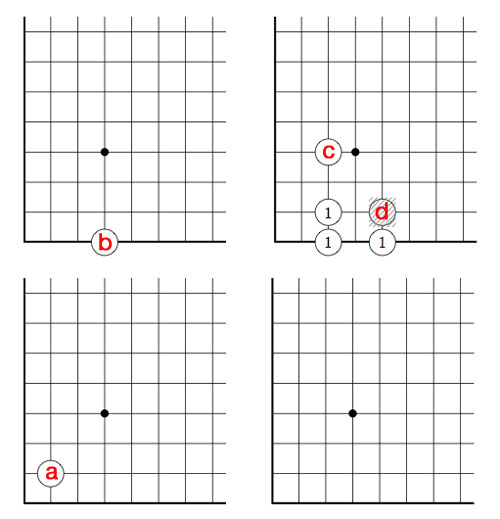Above is the unique winning move : we remove stone d and by the rectangle-rule add three new stones, marked 1. We only need to compute in finite fields to solve this and similar problems.

First note that the largest finite number occuring in a stone-coordinate is 4, so in this case we can perform all calculations in the field $\mathbb{F}_{2^{2^2}}(\omega) = \mathbb{F}_{2^{12}}$ where (as before) we identify $\mathbb{F}_{2^{2^2}} = { 0,1,2,\ldots,15 }$ and we have seen that $\omega^3=2$ (for ease of notation all Nim-additions and Nim-multiplications are denoted this time by + and x instead of $\oplus$ and $\otimes$ as we did last time, so for example $\omega^3 = \omega \otimes \omega \otimes \omega$).

If you’re not nimble with the Nim-tables, you can check all calculations in SAGE where we define this finite field via

sage: R.< x,y,z>=GF(2)[]
sage: S.< t,f,o>=R.quotient((x^2+x+1,y^2+y+x,z^3+x))

and we can now calculate in $\mathbb{F}_{2^{12}}$ using the symbols t for Two, f for Four and o for $\omega$. For example, we have seen that the nim-value of a stone is the nim-multiplication of its coordinates

sage: t*t
t + 1
sage: f*o
f*o
sage: (o+t)*(o+t+1)
o^2 + o + 1
sage: (o+f)*(o+1)
f*o + o^2 + f + o

That is, the nim-value of stone a is 3, stone b is $4 \times \omega$, stone c is $\omega^2+\omega+1$ and finally that of stone d is equal to $\omega^2+5 \times \omega +4$.

By adding them up, the nim-value of the original position is a finite number : 6. Being non-zero we know that the 2nd player has a winning strategy.

Just as in ordinary nim, we compare the value of a stone to the sum of the values of the other stones, to determine the stone we will play. These sums are for the four stones : 5 for a, $4 \times \omega+6$ for b, $\omega^2+\omega+7$ for c and $\omega^2+5 \times \omega+2$ for d. There is only one stone where this sum is smaller than the stone-value, so we know we have to make our move with stone d.

By the Nimbers-rule we need to find a rectangle with top-right hand corner $~(\omega+4,\omega+1)$ and lower-left hand corner $~(u,v)$ such that the values of the three new corners adds up to $\omega^2+5 \times \omega+2$, that is we have to solve

$u \times v + u \times (\omega+1) + (\omega+4)\times v = \omega^2+5 \times \omega+2$

where u and v are ordinals smaller than $\omega+4$ and $\omega+1$. u and v cannot be both finite, for then we wouldn’t obtain a $\omega^2$ term. Similarly (u,v) cannot be of the form $~(u,\omega)$ with u finite because then the left-hand sum would be $\omega^2+4 \times \omega + u$ and likewise it cannot be of the form $~(\omega+i,v)$ with i and v finite as then the coefficient of $\omega$ in the left-hand sum will be i+1 and we cannot take i equal to 4.

The only remaining possibility is that (u,v) is of the form $~(\omega+i,\omega)$ with i finite, in which case the left-hand sum equals $~\omega^2+ 5 \times \omega + i$ whence i=2 and we have found our unique winning move!

But, our opponent can make life difficult by forcing us to compute in larger and larger finite fields. For example, if she would move next by dropping the c stone down to the 256-th row, what would be our next move?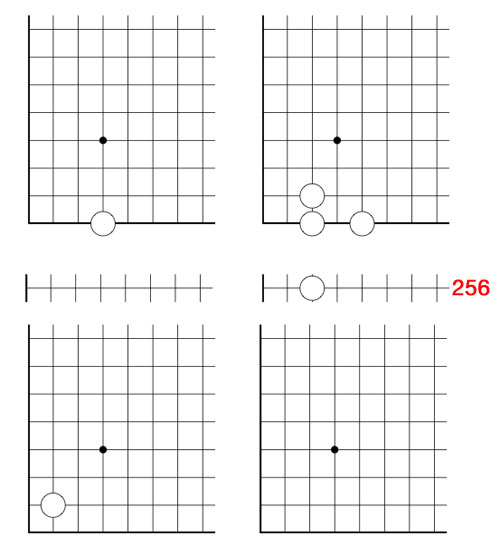(one possible winning move is to remove the stone at $~(\omega+2,\omega)$ and add stones at the three new corners of the rectangle : $~(257,2), (257,\omega)$ and $~(\omega+2,2)$)

Nimbers is a 2-person game, winnable only if you understand the arithmetic of the finite fields $\mathbb{F}_{2^{2^n}}$ associated to Fermat 2-powers.

It is played on a rectangular array (say a portion of a Go-board, for practical purposes) having a finite number of stones at distinct intersections. Here’s a typical position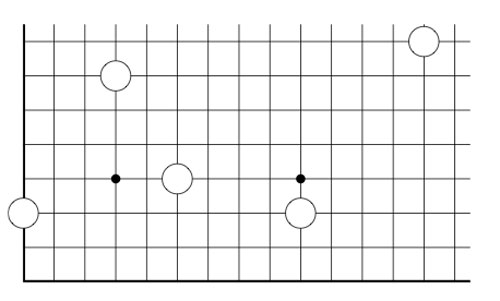The players alternate making a move, which is either

• removing one stone, or
• moving a stone to a spot on the same row (resp. the same column) strictly to the left (resp. strictly lower), and if there’s already a stone on this spot, both stones are removed, or
• adding stones to the empty corners of a rectangle having as its top-right hand corner a chosen stone and removing stones at the occupied corners

Here we illustrate two possible moves from the above position, in the first we add two new stones and remove 2 existing stones, in the second we add three new stones and remove only the top right-hand stone.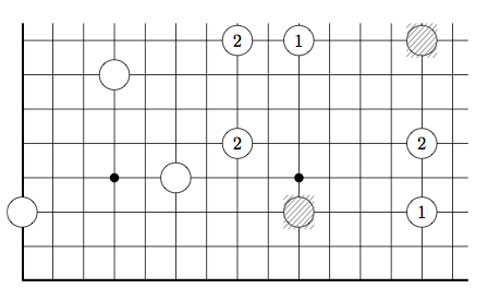As always, the last player able to move wins the game!

Note that Nimbers is equivalent to Lenstra’s ‘turning corners’-game (as introduced in his paper Nim-multiplication or mentioned in Winning Ways Chapter 14, page 473).

If all stones are placed on the left-most column (or on the bottom row) one quickly realizes that this game reduces to classical Nim with Nim-heap sizes corresponding to the stones (for example, the left-most stone corresponds to a heap of size 3).

Nim-addition $n \oplus m$ is defined inductively by

$n \oplus m = mex(n’ \oplus m,n \oplus m’)$

where $n’$ is any element of ${ 0,1,\ldots,n-1 }$ and $m’$ any element of ${ 0,1,\ldots,m-1 }$ and where ‘mex’ stands for Minimal EXcluded number, that is the smallest natural number which isn’t included in the set. Alternatively, one can compute $n \oplus m$ buy writing $n$ and $m$ in binary and add these binary numbers without carrying-over. It is well known that a winning strategy for Nim tries to shorten one Nim-heap such that the Nim-addition of the heap-sizes equals zero.

This allows us to play Nimber-endgames, that is, when all the stones have been moved to the left-column or the bottom row.

To evaluate general Nimber-positions it is best to add another row and column, the coordinate axes of the array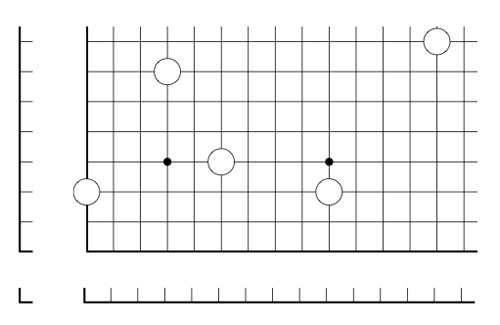and so our stones lie at positions (1,3), (4,7), (6,4), (10,3) and (14,8). In this way all legal moves follow the rectangle-rule when we allow rectangles to contain corners on the added coordinate axes. For example, removing a stone is achieved by taking a rectangle with two sides on the added axes, and, moving a stone to the left (or the bottom) is done by taking a rectangle with one side at the x-axes (resp. the y-axes)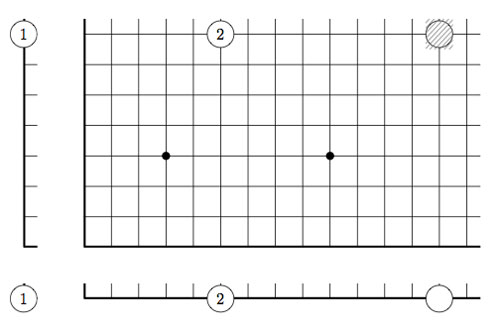However, the added stones on the coordinate axes are considered dead and may be removed from the game. This observation allows us to compute the Grundy number of a stone at position (m,n) to be

$G(m,n)=mex(G(m’,n’) \oplus G(m’,n) \oplus G(m,n’)~:~0 \leq m’ < m, 0 \leq n’ < n)$

and so by induction these Grundy numbers are equal to the Nim-multiplication $G(m,n) = m \otimes n$ where

$m \otimes n = mex(m’ \otimes n’ \oplus m’ \otimes n \oplus m \otimes n’~:~0 \leq m’ < m, 0 \leq n’ < n)$

Thus, we can evaluate any Nimbers-position with stone-coordinates smaller than $2^{2^n}$ by calculating in a finite field using the identification (as for example in the odd Knights of the round table-post) $\mathbb{F}_{2^{2^n}} = \{ 0,1,2,\ldots,2^{2^n}-1 \}$

For example, when all stones lie in a 15×15 grid (as in the example above), all calculations can be performed using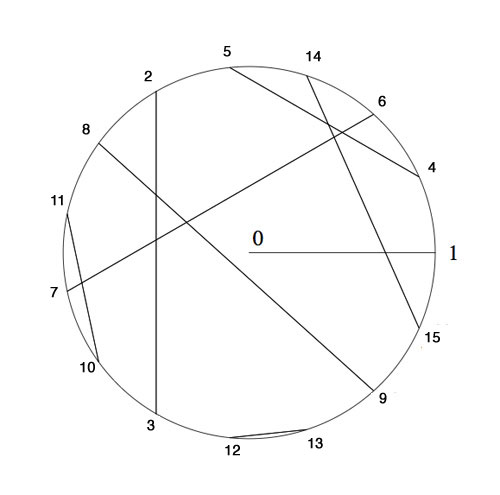Here, we’ve identified the non-zero elements of $\mathbb{F}_{16}$ with 15-th roots of unity, allowing us to multiply, and we’ve paired up couples $(n,n \oplus 1)$ allowing u to reduce nim-addition to nim-multiplication via

$n \oplus m = (n \otimes \frac{1}{m}) \otimes (m \oplus 1)$

In particular, the stone at position (14,8) is equivalent to a Nim-heap of size $14 \otimes 8=10$. The nim-value of the original position is equal to 8Suppose your opponent lets you add one extra stone along the diagonal if you allow her to start the game, where would you have to place it and be certain you will win the game?

The odd Knight of the round table problem asks for a consistent placement of the n-th Knight in the row at an odd root of unity, compatible with the two different realizations of the algebraic closure of the field with two elements.

The first identifies the multiplicative group of its non-zero elements with the group of all odd complex roots of unity, under complex multiplication. The second uses Conway’s ‘simplicity rules’ in ONAG to define an addition and multiplication on the set of all ordinal numbers.Here’s the seating arrangement for the first 15 knights. Conway proved that all finite ordinals smaller than $2^{2^i}$ form a subfield of $\overline{\mathbb{F}}_2$. The first non-trivial one being $\{ 0,1,2,3 \}$ with smallest multiplicative generator $2$, whence we place Knight 2 at $e^{2 i \pi/3}$ and as $2^2=3$ we know where to place the third Knight.

The next subfield is made of the numbers $\{ 0,1,2,\ldots,13,14,15 \}$ and its non-zero numbers form a cyclic group of order 15. Hence we need to find the smallest generator of this group satisfying the additional property of being compatible with the earlier seating, that is, its fifth power must equal to 2. Checking the multiplication table reproduced here one verifies that the wanted generator is 4 and so we place Knight 4 at $e^{\frac{2 \pi i}{15}}$ and as all the ordinals smaller than 16 are powers of 4 this tells us where to place the Knights until we get to the 15th in the row.

In february we were able to seat the first few thousand Knights by showing by hand that 32 is the smallest ordinal such that its 15-th power is equal to 4 and using SAGE that 1051 is the smallest ordinal whose 257-th power equals 32. These calculations enabled us to seat the Knights until we get to the 65536-th in the row.

Today I managed to show that 1361923 is the smallest ordinal such that its 65537-th power equals 1051. You can verify this statement in SAGE using the method explained in the february post

sage: R.< x,y,z,t,u >=GF(2)[]

sage: S.< a,b,c,d,e > =
R.quotient((x^2+x+1,y^2+y+x,z^2+z+x*y,t^2+t+x*y*z,u^2+u+x*y*z*t))

sage: (c*e+b*e+a*b*c*d+b*c*d+a*b*d+a+1)^65537
c^2 + b*d + a + 1

(It takes a bit longer to check minimality of 1361923). That is, we have to seat Knight 1361923 at $e^{\frac{2 \pi i}{4294967295}}$ and because all the numbers smaller than 4294967296 are powers of 1361923 we have seating arrangements for the first 4294967295 Knights!

I did try the same method in february but ran into time- and memory-problems on my 2.4Ghz 2Gb MacBook. Today I upgraded from Sage 3.3 to Sage 4.6 and this version is a lot faster (using the 64-bit architecture) and also appears to be much better at memory-management. Thank you, Sage-community!

Wishing you all a lot of mathematical (and other) fun in the prime-number year 2011.

atb :: lieven.